Question-and-Answer Resource for the Building Energy Modeling Community
Get started with the Help page

# People Definition : Carbon Dioxide Generation Rate Units???

I was looking for the carbon dioxide generation rate for an aerobics room. I got to the built in people definitions and they all use the same 0.000024 (IP Units) for the closed office, break room, and Secondary School Gym. Obviously i need to change this for a gym, so i look up Ashrae 62.1 2013 and find Appendix C Figure C2 which says maybe a 1 liter per minute. This is in SI units. So i try to convert to IP units but Open studio is not using CFM, it is using CFHR/MINBTU. Why does this unit include energy and not match Ashrae volumetric measurement. Also what is HR/MIN?

Can someone tell me the conversion?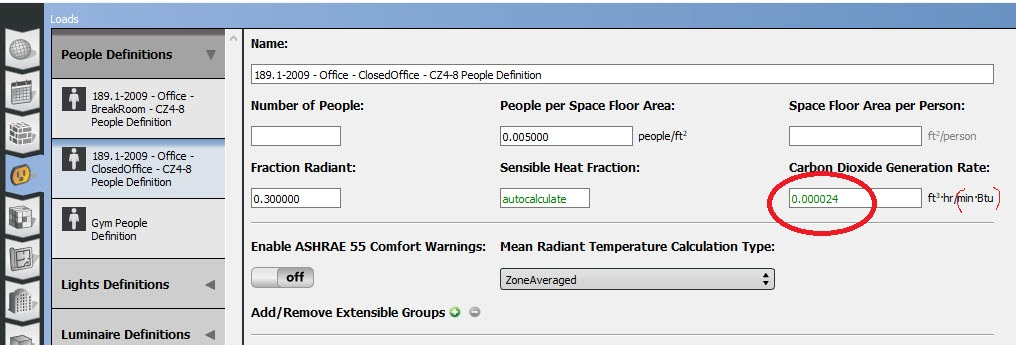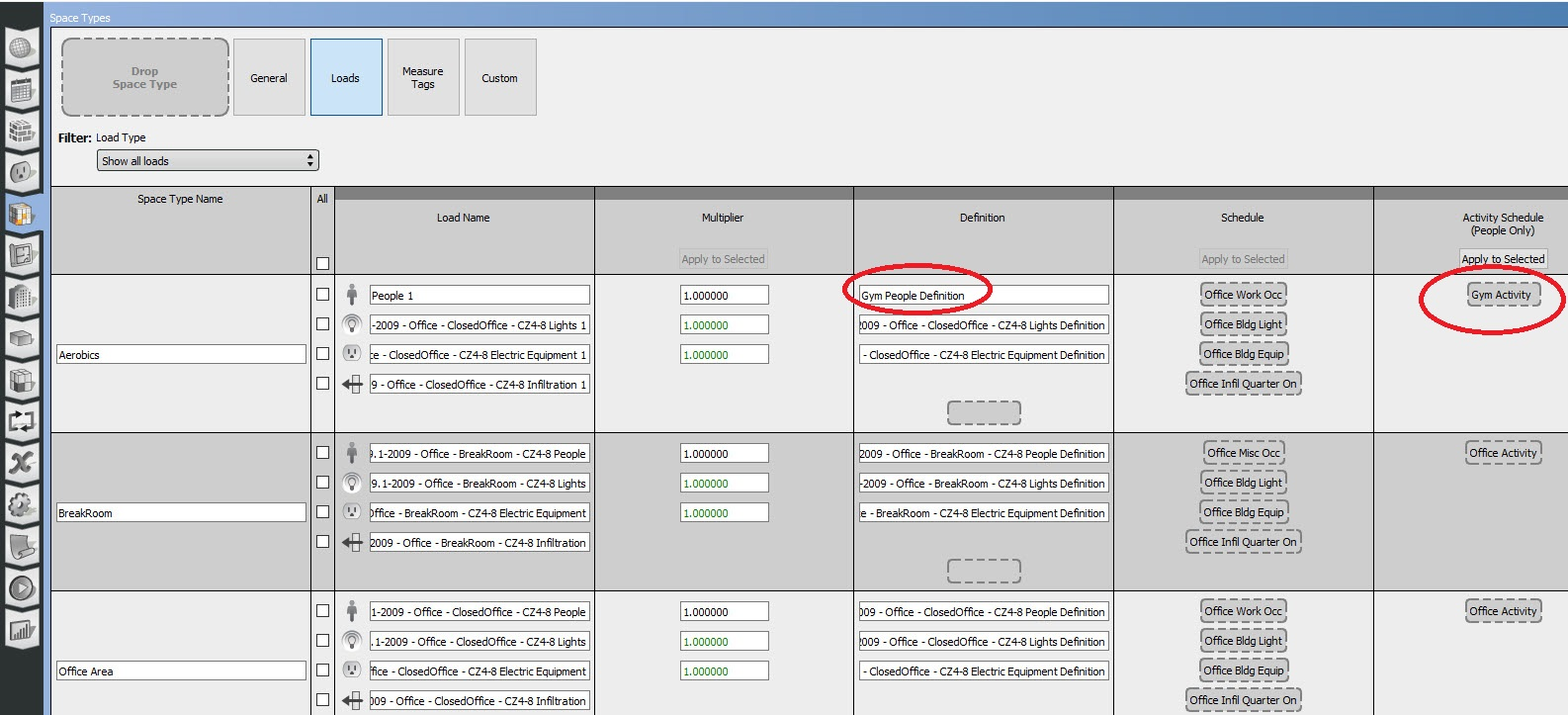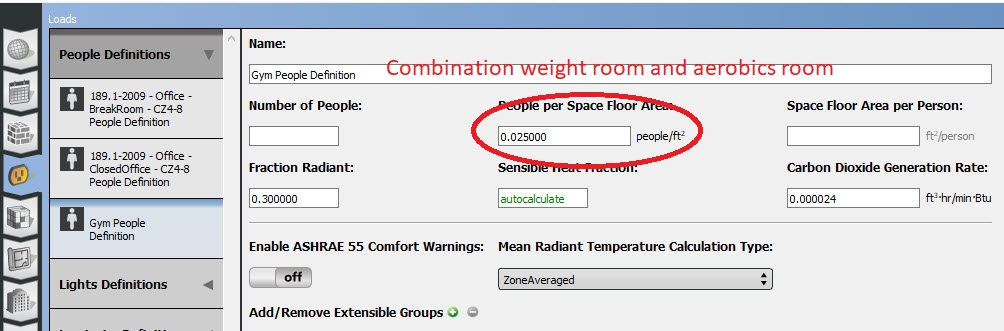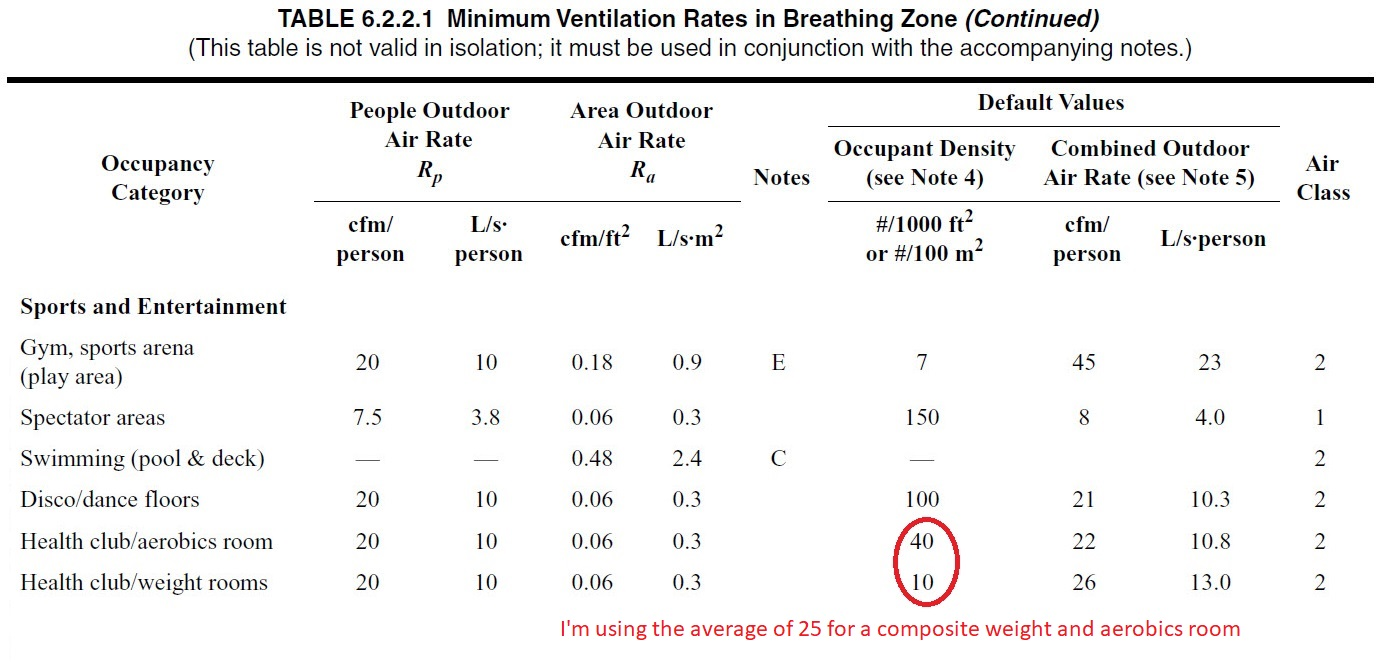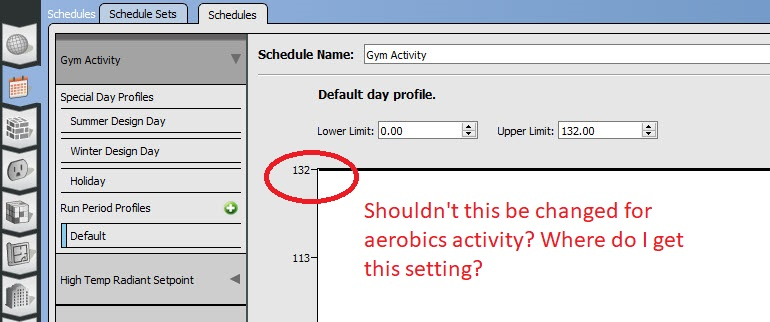edit retag close merge delete

Sort by » oldest newest most voted

TL;DR: According to ASHRAE 62.1, this input for carbon dioxide generation rate should be the same regardless of space type. That's why it is the same for all space types in OpenStudio library files. What you need to change between space types are the other input fields for occupants (activity schedule, occupancy schedule, and occupant density) so that EnergyPlus can properly calculate the volume of carbon dioxide generated.

Explanation

The SI unit for that carbon dioxide generation rate input input field is actually

$$\frac{L}{s*W}$$

so it's a volumetric rate of $CO_2$ divided by the activity rate of the occupants. That's why the IP unit translates to

$\frac{ft^3}{min} / \frac{Btu}{hr}$ = $\frac{ft^3*hr}{min*Btu}$

The ASHRAE 62.1-2013 figure that you mentioned is shown below. For the "maybe 1 liter per minute" of $CO_2$ generation that you referenced, I'm guessing that you are looking at the metabolic rate of ~4 met.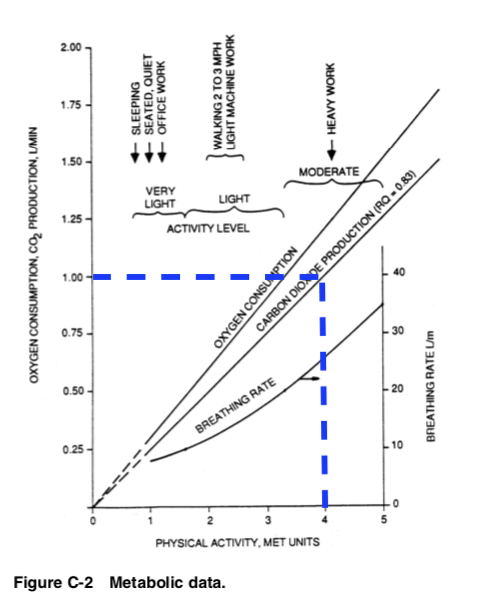That, however, is for one specific metabolic rate value. Note that the $CO_2$ generation curve is a straight line, so you can easily calculate volumetric $CO_2$ generation based on metabolic rate. This generation rate input that you're asking about is the slope of that curve. The default value is 3.82E-8 $\frac{m^3}{s*W}$ or 3.82E-5 $\frac{L}{s*W}$, which you can see in OpenStudio if you create a new People load definition. This default was obtained from ASHRAE Standard 62.1-2007 value at 0.0084 $\frac{cfm}{met*person}$ over the general adult population. Because it's a straight line, this generation rate input is the same no matter what space type you are simulating.

EnergyPlus uses the activity schedule, occupancy schedule, and occupant density input fields in order to calculate the physical activity of occupants (horizontal axis of the ASHRAE 62.1 figure). If you set these three inputs to reflect gym occupants, then you should get ~4 met. These inputs are then used with the generation rate input (slope of the line in the ASHRAE 62.1 figure) in order to calculate the volumetric $CO_2$ generation (vertical axis of the ASHRAE 62.1 figure). The schedules are assigned to space types in OpenStudio, and the occupant density is assigned to the people load definition (see below).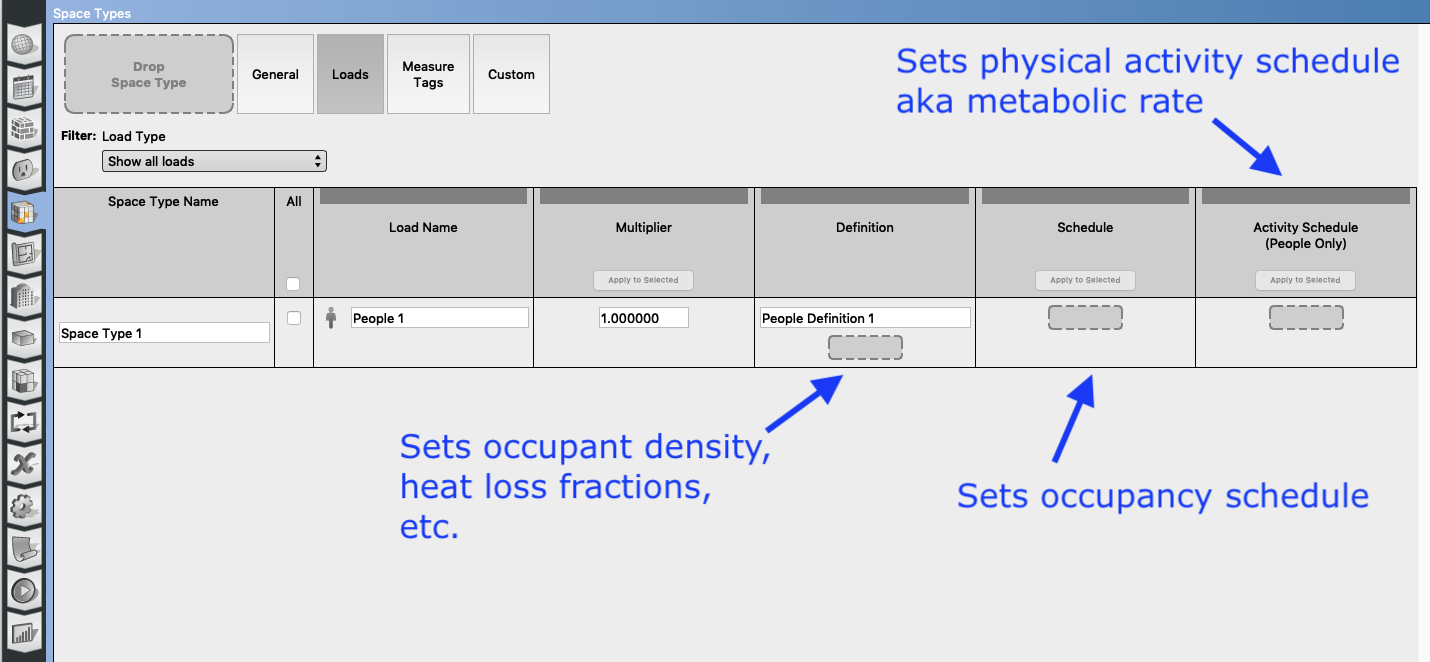UPDATE

The units that OpenStudio expects for the activity schedule are $\frac{W}{person}$. There is a table of metabolic rates for various activities in the EnergyPlus documentation for the People object. This has a range of 315 - 423 $\frac{W}{person}$ for "calisthenics/exercise", which is equivalent to 3 - 4 $met$ assuming each occupant has 1.8 $m^2$ of surface area. This table of metabolic rates can be found in ASHRAE Standard 55 (Table 5.2.1.2 in 55-2010).

more

So the Ashrae table is wrong? What is the conversion constant?

@TheEnergyTutor no, the input is a reflection of the line's slope from the ASHRAE figure. I've updated my answer to add more information.

The number i'm getting for a default is 0.000024 (1000CFM/MBH) per this screenshot. This is the number i am trying to adjust for my "Gym People Definition" I added a photo to the original post.

@TheEnergyTutor you don't adjust that generation rate value in the gym people definition. This value should be the same for all people definitions, according to ASHRAE 62.1. You adjust the occupant density in the gym people definition, as well as the activity schedule and occupancy schedule assigned to the gym space type. EnergyPus calculates the generated volume of $CO_2$ from the combination of these inputs. I've updated my answer to be clearer.

OK. The occupant density is per the table so that is easy, I just don't understand what to set the activity value to. I have uploaded more photos to show this clearer. I think i am close to understanding how to do this, but getting stuck on this last item.